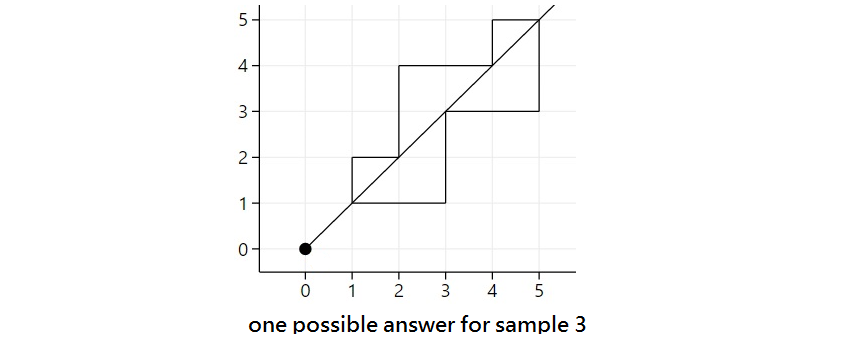시간 제한 메모리 제한 제출 정답 맞은 사람 정답 비율
1 초 512 MB 1 1 1 100.000%

## 문제

Consider the following game about splitting a simple polygon with $N$ vertices on a plane. The purpose of this game is using a straight line which passes through the origin to split the given simple polygon into as many non-zero area regions as possible. Please finish the game with the best result possible.

## 입력

The input consists of $N+1$ lines. The first line contains an integer $N$. The $i$-th of the following $N$ lines consists of two integers $x_i$ and $y_i$ indicating the vertices of the given polygon in counter-clockwise order.

## 출력

Output one integer: the maximum number of non-zero area regions into which the given polygon can be split by a single line passing through the origin.

## 제한

• $1 \le N \le 10^5$
• $1 \le x_i, y_i \le 10^9$
• if $i \ne j$, then $(x_i, y_i) \ne (x_j, y_j)$
• the vertices are given in counter-clockwise order

## 예제 입력 1

4
1 1
2 1
2 2
1 2


## 예제 출력 1

2


## 예제 입력 2

6
2 1
4 2
8 4
4 8
2 4
1 2


## 예제 출력 2

2


## 예제 입력 3

10
1 1
3 1
3 3
5 3
5 5
4 5
4 4
2 4
2 2
1 2


## 예제 출력 3

5


## 힌트